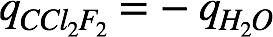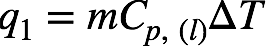# Problem: Compounds such as CCl2F2 are known as chlorofluorocarbons, or CFCs. These compounds were once widely used as refrigerants but are now being replaced by compounds that are believed to be less harmful to the environment. The heat of vaporization of CCl2F2 is 289 J/g.What mass of this substance must evaporate to freeze 210 g of water initially at 21 oC? (The heat of fusion of water is 334 J/g; the specific heat of water is 4.18 J/(g K).)

🤓 Based on our data, we think this question is relevant for Professor Thompson's class at SHSU.

###### FREE Expert Solution

The amount of heat released by water during it's freezing is absorbed by CCl2F2 for it to evaporate. This means:The process of freezing 210 g of water initially at 21 ˚C involves two quantities of heat:

q1 =  Heat decrease the temperature of water from 21 ˚C to 0˚C: Cp, (l) = 4.18 J/(g K).
q2 = Heat completely freeze the water: ΔH˚freezing = –ΔH˚fusion = –334 J/g.

For q1: This amount of heat is given by the equation:where m = mass of water, Cp, (l) = specific heat capacity of water, ΔT = Tfinal – Tinitial = change in temperature. We're given:

• m = 210 g
• Cp, (l) = 4.18 J/(g K)
• ΔT = 0 – 21 ˚C = –21 ˚C = –21 K###### Problem Details

Compounds such as CCl2F2 are known as chlorofluorocarbons, or CFCs. These compounds were once widely used as refrigerants but are now being replaced by compounds that are believed to be less harmful to the environment. The heat of vaporization of CCl2F2 is 289 J/g.

What mass of this substance must evaporate to freeze 210 g of water initially at 21 oC? (The heat of fusion of water is 334 J/g; the specific heat of water is 4.18 J/(g K).)